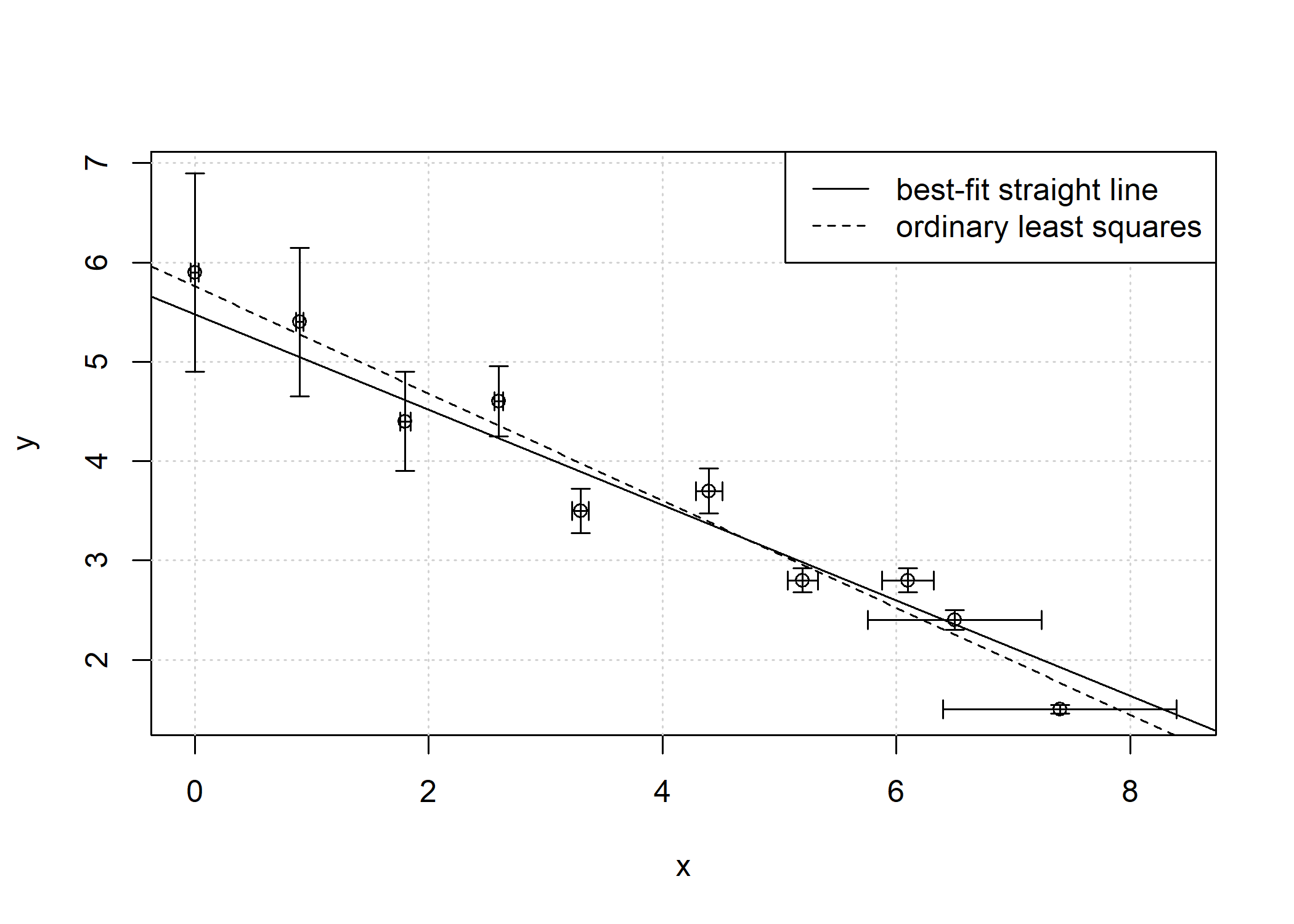# Best-Fit Straight Line

Provides the solution from York (1968) for fitting a straight line to bivariate data with errors in both coordinates. It gives unbiased estimates of the intercept, slope and standard errors of the best-fit straight line to independent points with (possibly correlated) normally distributed errors in both x and y. Other commonly used errors-in-variables methods, such as orthogonal distance regression, geometric mean regression or Deming regression are special cases of York’s solution.

# R package bfst: Best-fit Straight LineThe solution for the best-fit straight line to independent points with normally distributed errors in both x and y is known from York (1966, 1968, 2004). It provides unbiased estimates of the intercept, slope and standard errors of the best-fit straight line, even when the x and y errors are correlated.

Surprisingly, as Wehr and Saleska (2017) point out, York's solution has escaped the attention of many scientists that are writing on straight-line fitting with errors in both x and y (also known as Model II regressions, errors-in-variables models or measurement error models).

Other commonly used least-squares estimation methods, such as ordinary least-squares regression, orthogonal distance regression (also called major axis regression), geometric mean regression (also called reduced major axis or standardised major axis regression) or Deming regression are all special cases of York’s solution and only valid under particular measurement conditions.

The bfsl package implements York's general solution and provides the best-fit straight line of bivariate data with errors in both coordinates.

## Example## Release notes

See the NEWS file for latest release notes.

## References

York, D. (1966). Least-squares fitting of a straight line. Canadian Journal of Physics, 44(5), 1079–1086, https://doi.org/10.1139/p66-090

York, D. (1968). Least squares fitting of a straight line with correlated errors. Earth and Planetary Science Letters, 5, 320–324, https://doi.org/10.1016/S0012-821X(68)80059-7

York D. et al. (2004). Unified equations for the slope, intercept, and standard errors of the best straight line, American Journal of Physics, 72, 367-375, https://doi.org/10.1119/1.1632486

Wehr, R. and Saleska, S. R. (2017) The long-solved problem of the best-fit straight line: application to isotopic mixing lines, Biogeosciences, 14, 17-29, https://doi.org/10.5194/bg-14-17-2017

Initial release.

# Reference manual

install.packages("bfsl")

0.1.0 by Patrick Sturm, 2 years ago

https://github.com/pasturm/bfsl

Report a bug at https://github.com/pasturm/bfsl/issues

Browse source code at https://github.com/cran/bfsl

Authors: Patrick Sturm [aut, cre]

Documentation:   PDF Manual